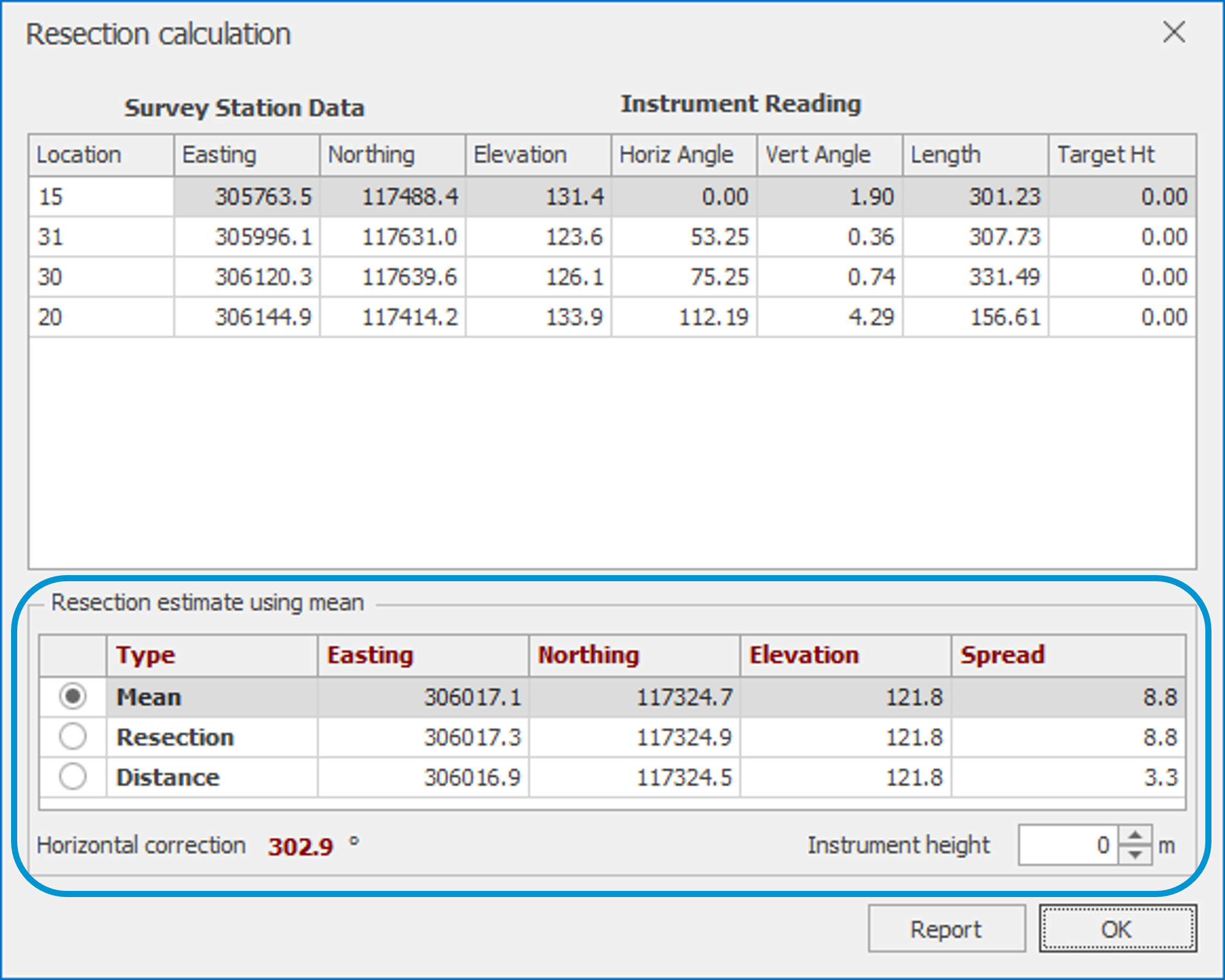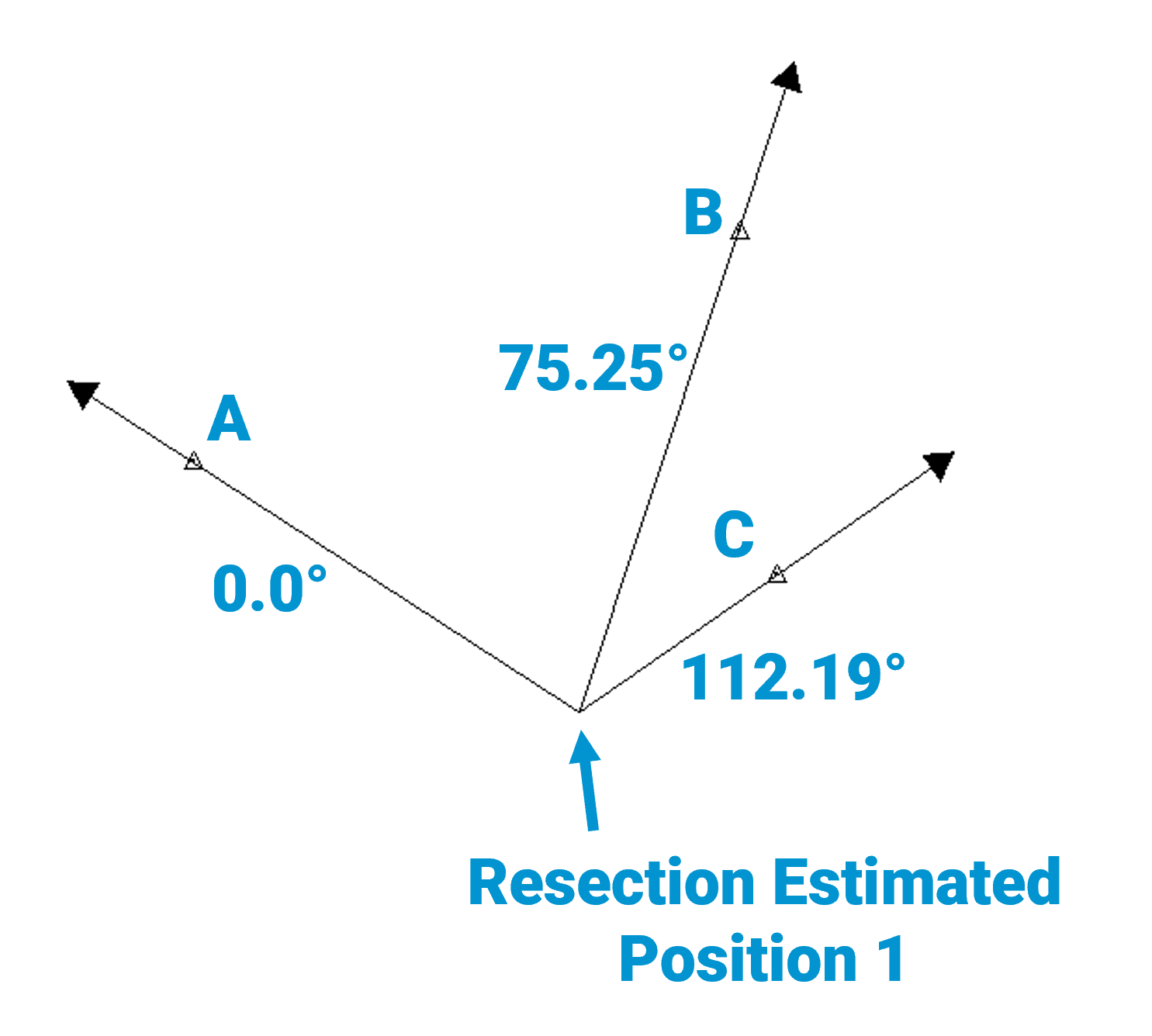# Survey Resections in SHOTPlus™SHOTPlus™ Variant:
Surface
Version:
6.1.1 and above
KBA Summary:
This KBA describes the different methods used for resection calculations when importing data collected by a Survey Instrument into SHOTPlus™.

### Introduction

When importing data collected by a Survey Instrument (laser scanner, total station or theodolite), into SHOTPlus™, the position of the Survey Instrument in three dimensional space must be defined.

If this position is unknown, it will be calculated by SHOTPlus™ using a resection. This Knowledge Base Article (KBA) describes the different methods used for the resection calculation and the information provided by SHOTPlus™.

### Method

To calculate the position of the Survey Instrument via resection, SHOTPlus™ uses the Horizontal Angle, Vertical Angle, and Distance between the Survey Instrument and four, or more, known points grouped into triplets. For example, known points A, B, C, and D would be grouped into the following triplets:

1. A, B and C;
2. B, C and D;
3. C, D, and A; and
4. D, A, and B.

A triangulation is completed with each set of triplets to produce an estimated location of the Survey Instrument:

1. Triangulation with A, B, and C triplet = Estimated Position 1;
2. Triangulation with B, C, and D triplet = Estimated Position 2;
3. Triangulation with C, D, and A triplet = Estimated Position 3; and
4. Triangulation with D, A, and B triplet = Estimated Position 4.

The mean of these estimated positions is used as the calculated position. Two different calculated positions, each triangulated using a different method, are reported by SHOTPlus™. These are; 'Distance', and 'Resection'. In addition, the mean of all 'Distance' and 'Resection' estimated position triangulations is reported as the 'Mean' calculated position.#### 'Distance' Triangulation

The 'Distance' Triangulation is completed by comparing the distance and vertical angle, between the imported Survey Instrument and the known points. The position of the imported Survey Instrument is calculated as the point where these distances overlap, as illustrated in the diagram below.

#### 'Resection' Triangulation

The 'Resection' Triangulation is completed by comparing the horizontal angle and vertical angle, between the imported Survey Instrument and the known points. The position of the imported Survey Instrument is calculated as the point where these angles overlap, as illustrated in the diagram below.A 'Spread' is reported for both the 'Distance' and 'Resection' triangulations to give an indication of the accuracy of the Survey Instrument's calculated position.

To calculate the spread, the estimated positions (calculated by either the Distance or Resection triangulations) are plotted in three dimensional space, and a three dimensional prism is fitted around the points. The reported spread value is equal to the greatest distance between two diagonally opposite points of this prism.

The spread reported for the 'Mean' calculated position is equal to the the spread calculated for both the 'Distance' and 'Resection' triangulations.#### Horizontal Correction

Horizontal correction describes the angle between the first Known Point collected by the Surveyor (recorded as 0.0°) and True North, as illustrated in the diagram below.